Home | | Chemistry 12th Std | Coordination Chemistry: Answer the following questions

# Coordination Chemistry: Answer the following questions

Chemistry : Coordination Chemistry: : Book Back Important Questions, Answers, Solutions : Answer briefly, Answer in detail, Exercise Numerical Problems

Coordination Chemistry

1. Write the IUPAC names for the following complexes.

i) Na2 [Ni( EDTA)]

ii) [Ag (CN)2]

iii) [Co(en)3]2 (SO4)3

iv) [Co(ONO) (NH3)5]2+

v) [Pt(NH3)2 Cl(NO2)]

Na2[Ni(EDTA)] : Sodium ethylenediaminetetraacetato nickelate (IV)

[Ag(CN)2] : Dicyanidoargentate (I) ion

[Co(en)3]2(SO4)3 : Tris (ethylenediamine) cobalt (III) sulphate

[CO(ONO)(NH3)5]2+ : pentaamminenitrito -κ -O - cobalt(III)ion

[Pt(NH3)2Cl2(NO2)] : Di ammine dichloridonitritoplatinum(II)

2. Write the formula for the following coordination compounds.

a) potassiumhexacyanidoferrate(II)

b) pentacarbonyliron(0)

c) pentaamminenitrito −κ −N -cobalt(III)ion

d) hexaamminecobalt(III)sulphate

e) sodiumtetrafluoridodihydroxidochromate(III)

a) Potassiumhexacyanidoferrate (II) - Formula : K4[Fe(CN)6]

b) Pentacarbonyliron(0) - Formula : [Fe(CO)5]

c) Pentaamminenitrito - κ -N - cobalt(III)ion - Formula : [Co(NH3)5(NO2)]2+

d) Hexaamminecobalt (III) sulphate - Formula : [Co(NH3)6]SO4

e) Sodium tetrafluoridodihydroxido chromate(III) - Formula : Na3[CrF4(OH)2]

3. Arrange the following in order of increasing molar conductivity

i) Mg [Cr (NH3) (Cl)5 ]

ii) [ Cr (NH3)5 Cl ]3 [CoF6]2

iii) [Cr (NH3)3 Cl3 ]

Answer: c < a < b

(c) does not gives any ion in solution, (a) gives Mg2+ and [Cr(NH3)Cl5]2− ion solution. Hence conductivity higher than (a), (b) gives 5 ions in solution. Hence molar conductivity of (b) is greater than other two. Hence order is c< a < b

4. Ni2+ is identified using alcoholic solution of dimethyl glyoxime. Write the structural formula for the rosy red precipitate of a complex formed in the reaction.5. [CuCl4 ]2 exists while [CuI4 ]2 does not exist why?

Size of I− is greater than Cl. Due to steric effect [CuI4]2− does not exist, I−  is also get oxidized to iodine.

6.   Calculate the ratio of [Ag+]/[Ag(NH3)2] in 0.2 M solution of NH3. If the stability constant for the complex [Ag(NH3)2] is 1 7 x 107.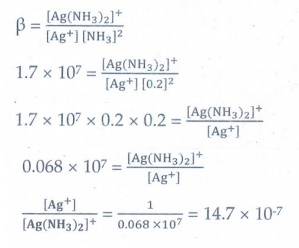β = [Ag(NH3)2]+ / [Ag+][NH3]2

1.7 × 107 = [Ag(NH3)2]+ / [Ag+][0.2]2

1.7 × 107 × 0.2 × 0.2 = [Ag(NH3)2]+ / [Ag+]

0.068 × 107 = [Ag(NH3)2]+  / [Ag+]

[Ag+] / [Ag(NH3)2]= 1 / [ 0.068 × 107] = 14.7 × 10-7

7. Give an example of coordination compound used in medicine and two examples of biologically important coordination compounds.

Medicine

(2) Cis-platin - Antitumor drug in cancer treatment.

Biologically important coordination compounds

(i) Fe2+ − Porphyrin complex carrying oxygen from lungs to tissues and carbon dioxide from tissues to lungs.

ii) Chlorophyll - A coordination complex containing Mg2+, Important role in photosynthesis, by which plants converts CO2 and water into carbohydrates and oxygen.

8. Based on VB theory explain why [Cr (NH3)6]3+ is paramagnetic, while [Ni (CN)4]2- diamagnetic9. Draw all possible geometrical isomers of the complex [Co( en)Cl2]and identify the optically active isomer.

[Co(en)2Cl2]+ and its optically active isomer10. [Ti ( H2O)6]3+ is coloured, while [Sc ( H2O)6 ]3+ is colourless- explain.

Ti: [Ar]3d24s2           Sc: [Ar]3d14s2

[Ti(H2O)6]3+              [Sc(H2O)6]3+

Ti3+: [Ar]3d14s0          Sc3+: [Ar]3d°4s°

One d electron present in [Ti(H2O)6]3+. Due to d-d transition the complex is coloured. In [Sc(H2O)6]3+ there is no d electron. Hence d-d transition is not possible in [Sc(H2O)6]3+ Hence it is colorless.

11. Give an example for complex of the type [Ma2 b2c2 ] where a, b, c are monodentate ligands and give the possible isomers.

Example for [Ma2b2C2] is [CoCl2 Br2(NH3)2]2−

5 geometrical isomers possible for this type complex.12. Give one test to differentiate [ Co (NH3)5Cl] SO4 and [ Co( NH3)5 SO4 ] Cl.

The complexes show ionization isomerism.

If SO42− ion is counter ion then the complex is [CO(NH3)5Cl]SO4. With BaCl2 it gives curdy white precipitate.

If Cl ion is counter ion then the complex [Co(NH3)5SO4]Cl. With silver nitrate it gives white precipitate.

13. In an octahedral crystal field, draw the figure to show splitting of d orbitals.

During crystal field splitting in octahedral field, in order to maintain the average energy of the orbitals (barycentre) constant, the energy of the orbitals dx2−y2 and dz2 (represented as eg orbitals) will increase by 3/5∆o while that of the other three orbitals dxy, dyz and dzx (represented as t2g orbitals) decrease by 2/5∆o. Here, Δo represents the crystal field splitting energy in the octahedral field.14. What is linkage isomerism? Explain with an example.

When an ambidentate ligand is bonded to the central metal atom / ion through either of its two different donor atoms gives linkage isomerism

Example: [Co(NH3)5(NO2)]2+ [Co(NH3)5(ONO)]2+

The nitrite ion is bound to the central metal ion Co3+ through a nitrogen atom in one complex, and through oxygen atom in other complex.

15. Write briefly about the applications of coordination compounds in volumetric analysis.

1. EDTA is used as a chelating ligand for the separation of lanthanides, in softening of hard water and also in removing lead poisoning.

3. Some metal ions are estimated by complex formation. Ni2+ ions estimated as [Ni(DMG)2]

3. Coordination complexes are used in the extraction of silver and gold from their ores by forming soluble cyanido complex. These cyanido complexes are reduced by zinc to yield metals. This process is called as Mac- Arthur -Forrest cyanide process.

16. Classify the following ligand based on the number of donor atoms.

a) NH3 b) en c) ox2- d) triaminotriethylamine e) pyridine

a) NH3 - Monodentate

b) en - Bidentate

c) ox2− - Bidentate

d) triaminotriethylamine - Tridentate

e) pyridine - Monodentate

17. Give the difference between double salts and coordination compounds.Double salt

• The ions present in the constituents are obtained when dissolved in water

• It does not completely lose its identity in solution

• The properties of the double salt are nearly the same as those of the constituent compounds

• Example: FeSO4.(NH4)2SO4.6H2O

Coordination compound

• Some ions present in the constituents are not obtained when dissolved in water

• It loses its identity in solution

• The properties of a coordination compound are usually different from those of the constituent compounds

• Example: K4[Fe(CN)6]

18. Write the postulates of Werner’s theory.

1. Most of the elements exhibit, two types of valence namely primary valence and secondary valence and each element tend to satisfy both the valences.

2. The primary valence: It is positive in most of the cases and zero in certain cases. They are always satisfied by negative ions. It is referred as the oxidation states.

3. The secondary valence: It is satisfied by negative ions, neutral molecules, positive ions or the combination of these. It is referred as the coordination number.

4. The inner sphere is known as coordination sphere and the groups present in this sphere is attached to metal. The outer sphere is called ionisation sphere and it can be separated into ions in a suitable solvent.

5. The primary valences are non-directional while the secondary valences are directional. The geometry of the complex is determined by the secondary valence.

19. [Ni ( CN)4 ]2 is diamagnetic, while [ NiCl4 ]2 is paramagnetic , explain using crystal field theory.

Ni: [Ar]3d8 4s2

Oxidation state of Ni in the complex = +2

Ni2+ : [Ar]3d8 4s0

CN is strong ligand. The complex is square planar.

Crystal field configurationThere is no unpaired electron in square planar crystal field configuration.

µs= 0. Hence it is diamagnetic.

[NiCl4]2− is paramagnetic

Ni : [Ar]3d84s2

Oxidation state of Ni in the complex = +2

Ni2+ : [Ar]3d8 4s0

Cl is weak ligand. The complex is tetrahedral.

Crystal field configuration of the complex = e4t24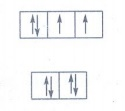Number of unpaired electron = 2

µs = √[n(n + 2)] = 2.8 BM

20. Why tetrahedral complexes do not exhibit geometrical isomerism.

All the ligands are adjacent to one another in tetrahedral complexes. Hence tetrahedral complexes do not exhibit geometrical isomerism.

21. Explain optical isomerism in coordination compounds with an example.

Optical Isomerism

Coordination compounds which possess chirality exhibit optical isomerism.

The pair of two optically active isomers which are mirror images of each other are called enantiomers.

The solutions rotate the plane of the plane polarised light to clockwise are called 'd' isomers (dextro rotatory).

The solutions rotate the plane polarised light to anticlockwise are called ‘l’ isomers (laevo rotatory).

The octahedral complexes of type [M(xx)3], [M(xx)2AB] and [M(xx)2B2] exhibit optical isomerism.

Examples: The optical isomers of [CoCl2(en)2]+22. What are hydrate isomers? Explain with an example.

The exchange of free solvent molecules in the crystal lattice with a ligand in the coordination entity will give different isomers. These types of isomers are called solvate isomers.

If the solvent molecule is water, then these isomers are called hydrate isomers.

Example: CrCl3.6H2O has three hydrate isomers.

[Cr(H2O)6]Cl3 - Violet color: Gives three chloride ions in solution

[Cr(H2O)5Cl]Cl2.H2O - Pale green color: Gives two chloride ions in solution

[Cr(H2O)4Cl2]Cl.2H2O - Dark green color: Gives one chloride ion in solution

23. What is crystal field splitting energy?

When ligands approach the central metal ion, the d-orbitals split into two sets, one with lower energy and other with higher energy. The difference of energy between the two sets of orbitals is called cryatal field splitting energy.

24. What is crystal field stabilization energy (CFSE) ?

The crystal field stabilisation energy is defined as the energy difference of electronic configurations in the ligand field (ELF) and the isotropic field / barycentre (EiSO).

CFSE(∆Eo) = {ELF} – {Eiso}

= {[n t2g(-0.4) + n eg (0.6)] ∆o + npP} - {n’p P}

n t2g is the number of electrons in t2g orbitals

n eg is number of electrons in eg orbitals

nP is number of electron pairs in the ligand field

n'P is the number of electron pairs in the isotropic field (barycentre)

25. A solution of [Ni (H2O)6]2+ is green, whereas a solution of [Ni (CN)4 ]2+  is colorless - Explain

The complex [Ni(H2O)6]2+ is octahedral and it has two unpaired electron in its eg orbital. These unpaired electrons involves d-d transition and responsible for the green colour of the complex.

The complex [Ni(CN)4]2+ is square planar, all the electrons are getting paired and there is no availability of unpaired electrons for d-d transition. Due to the absence of unpaired electron [Ni(CN)4]2+ is colourless

26. Discuss briefly the nature of bonding in metal carbonyls.

M-CO σ bonding

There is an electron pair donation from the carbon atom of carbonyl ligand into a vacant d-orbital of central metal atom forms

M ← CO sigma bond.π-back bonding:

This sigma bond formation increases the electron density in metal d orbitals and makes the metal electron rich. To compensate for this increased electron density, a filled metal d-orbital interacts with the empty π* orbital on the carbonyl ligand and transfers the added electron density back to the ligand is called π-back bonding.

Synergic effect:

Thus in metal carbonyls, electron density moves from ligand to metal through sigma bonding and from metal to ligand through pi bonding, this synergic effect accounts for strong M ← CO bond in metal carbonyls.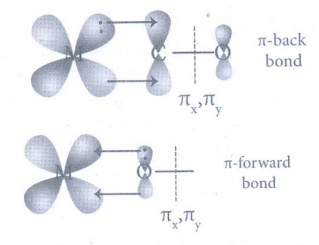27. What is the coordination entity formed when excess of liquid ammonia is added to an aqueous solution of copper sulphate?

When excess of liquid ammonia is added to an aqueous solution copper sulphate it forms a tetraamminecopper complex [Cu(NH3)4]SO4 (Tetraamminecopper(II) sulphate).

CuSO4 + 4NH3 → [Cu(NH3)4]SO4

The coordination entity is [Cu(NH3)4]2+

28. On the basis of VB theory explain the nature of bonding in [Co(C204)3]3-

Complex : [Co(C2O4)3]3-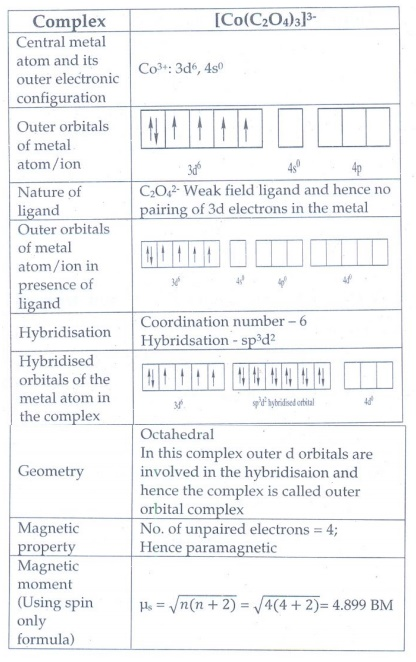29. What are the limitations of VB theory?

1. It does not explain the colour of the complex

2. It consider only the spin only magnetic moments and does not consider the other components of magnetic moments.

3. It does not provide a quantitative explanation inner orbital complexes and the others are outer orbital complexes for the same metal.

For example, [Fe(CN)6]4- is diamagnetic (low spin) whereas [FeF6]4- is paramagnetic (high spin). VB theory does not gives any explanation of this magnetic behavior.

30. Write the oxidation state, coordination number , nature of ligand, magnetic property and electronic configuration in octahedral crystal field for the complex K4[Mn (CN)6].

Oxidation state : +2

Coordination Number : 6

Nature of ligand : Strong field ligand

Magnetic property : Only one paired electron. µs = 1.732 BM

Electronic configuration in octahedral crystal field : t2g5 eg0

EVALUATE YOURSELF:

1. When a coordination compound CrCl3.4H2O is mixed with silver nitrate solution, one mole of silver chloride is precipitated per mole of the compound. There are no free solvent molecules in that compound. Assign the secondary valence to the metal and write the structural formula of the compound.

Solution:

CrCl3.4H2O shows hydrate isomerism. The complex is mixed with silver nitrate solution; one mole of silver chloride is precipitated per mole of the compound. There are no free solvent molecules in that compound.

Based on the above information it is concluded that one Cl ion present as ionisable valence. All the water molecules are present inside the coordination sphere. The complex may be [Cr(H2O)4Cl2]Cl

2. In the complex, [Pt(NO2)(H2O)(NH3)2Br , identify the following

i. Central metal atom/ion

ii. Ligand(s) and their types

iii. Coordination entity

iv. Oxidation number of the central metal ion

v. Coordination number

Complex : [Pt(NO2)(H2O)(NH3)2]Br

i. Central metal atom / ion : Pt / Pt+2

ii. Ligand(s) and their types : NO2 - Anioinic ligand, Monodentate

H2O - Neutral ligand, Monodentate

NH3 - Neutral ligand, Monodentate

iii. Coordination entity: [Pt(NO2)(H2O)(NH3)2]+

iv. Oxidation number of the central metal ion: +2

v. Coordination number: 4

3. A solution of [Co(NH3)4I2Cl when treated with AgNO3 gives a white precipitate. What should be the formula of isomer of the dissolved complex that gives yellow precipitate with AgNO3. What are the above isomers called?

A solution of [Co(NH3)4I2]Cl when treated with AgNO3 the Cl ion present outside of the coordination sphere gives a white precipitate.

If I is present outside of the coordination sphere it will gives yellow precipitate when treated with AgNO3. The formula is [Co(NH3)4(Cl)(I)]I. The isomers is called ionization isomer.

4. Write the IUPAC name for the following compounds

i) K2[Fe(CN)3(Cl)2(NH3)]

Potassium ammine dichlorido tri cyanido ferrate (III)

ii) [Cr(CN)2(H2O)4][Co(ox)2(en)]

(ethylene diamine) dioxalato cobalt (II)

iii) [CU(NH3)2Cl2]

Diammine dichlorido copper (II)

iv) [Cr(NH3)3(NC)2(H2O)]+

Triammine aqua dicyanido-κ Nchromium (III) ion

v) [Fe(CN)6]4

Hexacyanidoferrate (II) ion

5. Give the structure for the following compounds.

(i) Diammine silver(I) dicyanideargentate(I)

[Ag(NH3)2] [Ag(CN)2]

(ii) Pentaammine nitrito-κNcobalt (III) ion

[CO(NH3)5(NO2)]+2

(iii) Hexafluorido cobaltate (III) ion

[COF6]3-

(iv) Dichloridobis (ethylenediamine) cobalt (III) sulphate

[Co Cl2(en)2] SO4

(v) Tetra carbonyl nickel (0)

[Ni(CO)4]

6. Three compounds A, B and C have the molecular formula CrCl3.6H2O. They are kept in a container with a dehydrating agent and they lost water and attaining constant weight as shown below.Compond A : [Cr(H2O)4Cl2]Cl. 2H2O

Compound B : [Cr(H2O)5Cl]Cl2. H2O

Compound C : [Cr(H2O)3Cl3] . 3H2O

7. Indicate the possible type of isomerism for the following complexes and draw their isomers

(i) [Co(en)3][Cr(CN)6]

(ii) [Co(NH3)5(NO2)]2+

(iii) [Pt(NH3)3(NO2)]Cl

(i) [Co(en)3][Cr(CN)6] - Coordination isomerism

(iii) [Pt(NH3)3(NO2)]Cl - Ionisation isomerism

8. The spin only magnetic moment of Tetra chloride manganite (II) ion is 5.9 BM. On the basis of VBT, predict the type of hybridisation and geometry of the compound.

Tetra chloridomanganate (II) ion [MnCl4]2−

Coordination number of the complex is 4

It may be either tetrahedral or square planar.

Magnetic moment of the complex 5.9 BM shows that the complex must be a tetrahedral.

9. Predict the number of unpaired electrons in [CoCl4]2− ion on the basis of VBT.10. A metal complex having composition [Co(en)2Cl2]Br has been isolated in two forms A and B. (B) reacted with silver nitrate to give a white precipitate readily soluble in ammonium hydroxide. Whereas A gives a pale yellow precipitate. Write the formula of A and B. State the hybridization of Co in each and calculate their spin only magnetic moment.

Complex having two portion namely ionisable valence and coordination sphere. Ionisable valence only undergo reaction with the added reagent.

A given metal complex [Co(en)2Cl2]Br shows ionization isomerism and exhibits as [Co(en)2Cl2]Br or [Co(en)2ClBr]Cl.

[Co(en)2Cl2]Br gives pale yellow precipitate with silver nitrate because it ionizes to give [Co(en)2Cl2]+ and Br ion. Bromide ion gives pale yellow precipitate with silver nitrate. Hence A is [Co(en)2Cl2]Br

[Co(en)2ClBr]Cl gives white precipitate with silver nitrate because it ionizes to give [Co(en)2ClBr]+ and Cl- ion. Chloride ion gives white precipitate with silver nitrate and the precipitate is readily soluble in ammonium hydroxide. Hence B is [Co(en)2ClBr]Cl

A: [Co(en)2Cl2]Br

B: [Co(en)2ClBr]Cl

11. Draw all possible stereo isomers of a complex Ca[Co(NH3)Cl(Ox)2]

Ca[Co(NH3)Cl(Ox)2] shows both geometrical and optical isomerism

Geometrical isomerismCis isomer shows optical isomerism.12. The mean pairing energy and octahedral field splitting energy of [Mn(CN)6]3- are 28,800 cm−1 and 38500 cm−1 respectively. Whether this complex is stable in low spin or high spin?

Pairing energy of the complex (P) = 28,800 cm−1

Octahedral field splitting energy of the complex (∆o) = 38,500 cm−1

[Mn(CN)6]3−

Oxidation state = 3+

d configuration = d4

Shape = Octahedral

For high spin complex

Crystal field configuration = t2g3 eg1

CFSE (∆EO)

= {[n t2g(-0.4) + n eg (0.6)] ∆o +np P} - {n'p P}

n t2g = 3

n eg = 1

nP =0

n'P = 0

CFSE (∆EO) = {[3(-0.4) + 1(0.6)] ∆o + 0P} − 0

= [−1.2+ 0.6] ∆o = − 0.6 ∆o

= −0.6 × 38500 = 23100 cm−1

CFSE (∆EO) for high spin complex = 23100 cm−1

For low spin complex

Crystal field configuration = t2g4 eg0

CFSE (∆EO)

= {[n t2g(-0.4) + n eg (0.6)] ∆o + np P} – {n'p P}

n t2g = 4

n eg = 0

nP =1

n'P = 0

CFSE (∆EO) = {[4(-0.4) + 1(0.6)] Δo + 2P} − 0

= −1.6 ∆o + 1P

= (−1.6 × 38500) + (1 × 28800)

= −61600 + 28800 = −32800 cm−1

CFSE (∆EO) for low spin complex = −32800 cm−1

More negative CFSE value indicates that low spin complex is favoured.

The complex is stable in low spin.

13. Draw energy level diagram and indicate the number of electrons in each level for the complex [Cu(H2O)6]2+. Whether the complex is paramagnetic or diamagnetic?

[Cu(H2O)6]2+.

Oxidation state = 2+

d configuration = d9

Shape = Octahedral

Crystal field configuration = t2g6 eg3

Energy level diagramThe complex has one unpaired electron. Hence  it is paramagnetic. µB = 1.73 BM

14. For the [CoF6]3- ion the mean pairing energy is found to be 21000 cm−1. The magnitude of ∆0 is 13000 cm−1. Calculate the crystal field stabilization energy for this complex ion corresponding to low spin and high spin states.

[CoF6]3− ion

Oxidation state = 3+

d configuration = d6

Shape = Octahedral

Mean pairing energy = 21000 cm−1

Magnitude of Δ0 = 13000 cm−1

For high spin complex

Crystal field configuration = t2g4 eg2

CFSE (∆EO)

= {[n t2g(-0.4) + n eg (0.6)] ∆o + npP} − {n'p P}

n t2g = 4

n eg = 2

nP =1

n'P = 0

CFSE (∆Eo)

= {[4(-0.4) + 2(0.6)] ∆o + 1P} − 0

= [−1.6+ 1.2] ∆o + 1P = −0.4 Δo + 1P

= −0.4 × 13000 + 1 ×21000 = −5200 + 21000

= 15800 cm−1

CFSE (∆EO) for high spin complex = 15800 cm−1

For low spin complex

Crystal field configuration = t2g6 eg0

CFSE (∆EO)

= {[n t2g(−0.4) + n eg (0.6)] ∆o + np P} − {n'p P}

n t2g = 6

n eg = 0

nP = 3

n'P = 0

CFSE (∆E0)

= {[6(-0.4) + 0(0.6)] ∆o + 3P} − 0

= − 2.4 ∆o + 3P

= −2.4×13000 + 3×21000 = − 31200 + 63000

= 31800 cm−1

CFSE (∆EO) for low spin complex = 31800 cm−1

High positive value observed for low spin complex. It indicates that low spin complex is not favorable.

The complex is stable in high spin.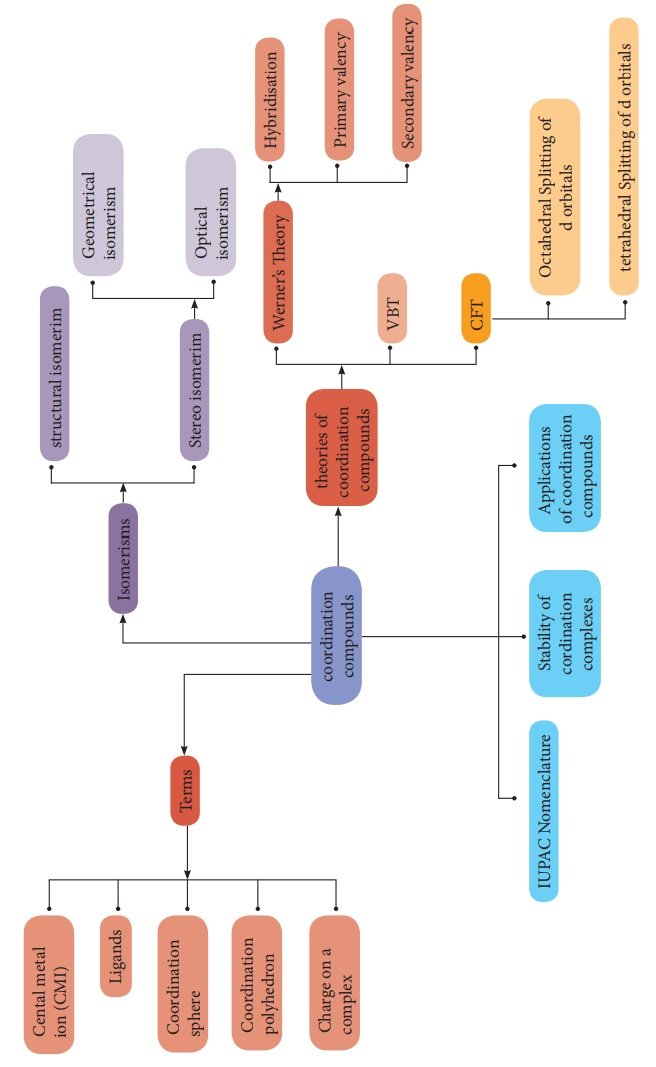Tags : Book Back and Important Questions Answers , 12th Chemistry : UNIT 5 : Coordination Chemistry
Study Material, Lecturing Notes, Assignment, Reference, Wiki description explanation, brief detail
12th Chemistry : UNIT 5 : Coordination Chemistry : Coordination Chemistry: Answer the following questions | Book Back and Important Questions Answers